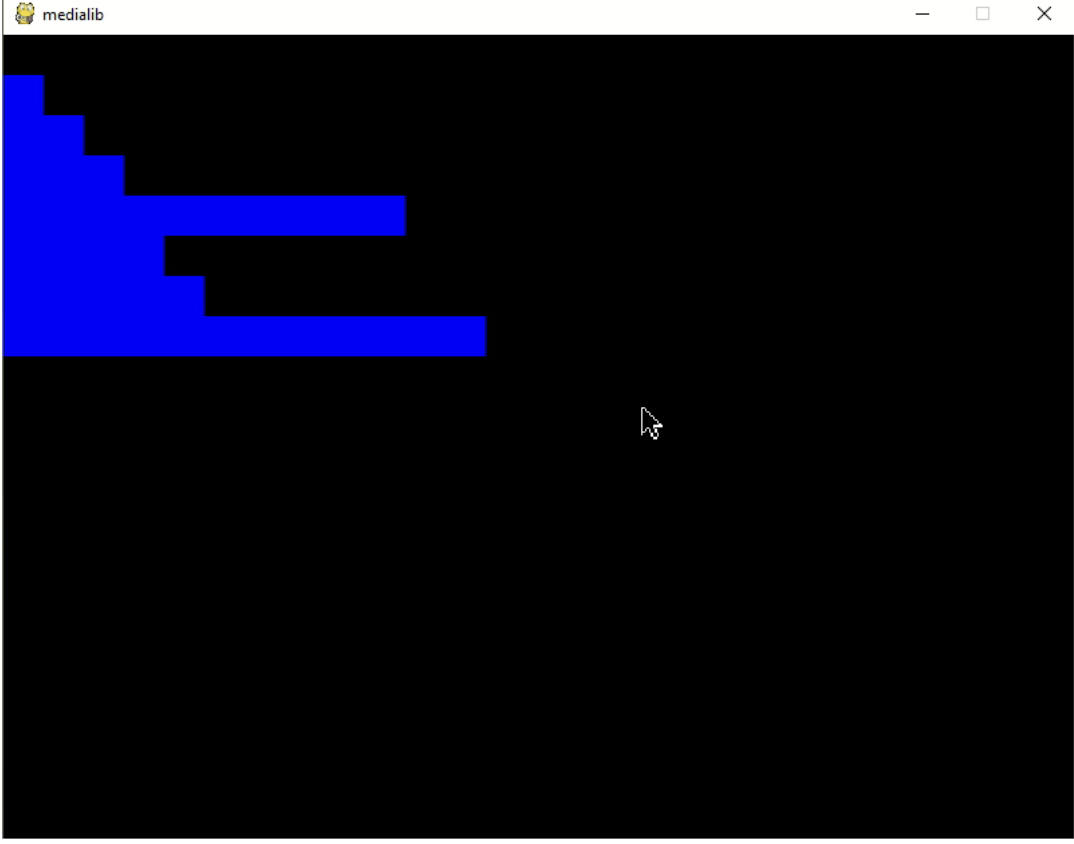## Tutorial

### Introduction

This tutorial was designed for Master’s Students who don’t have programming experience and contains exercises related to Medialib with not too difficult maths content. There are 3 hours of practical content suggested being used across two sessions.

Currently, Medialib is used in the Introduction to Computer Science Module for MSc Business Analytics (since 2021) and Master of Data Science (since 2022) at Durham University.

All of the examples in this tutorial can be downloaded from the Gallery.

### Practice 1

#### Practice 1-1

##### Learning Objectives

We are going to learn:

1. How to use the function defined by other's library.
2. Some void functions defined in medialib.
##### Introduction to MediaLib

First, let's draw some images:

• Open the program example.ipynb/example.py.
• Can you “read” this program?
• What do you think happens in line 7?
• And in line 9?
• What happens in line 11?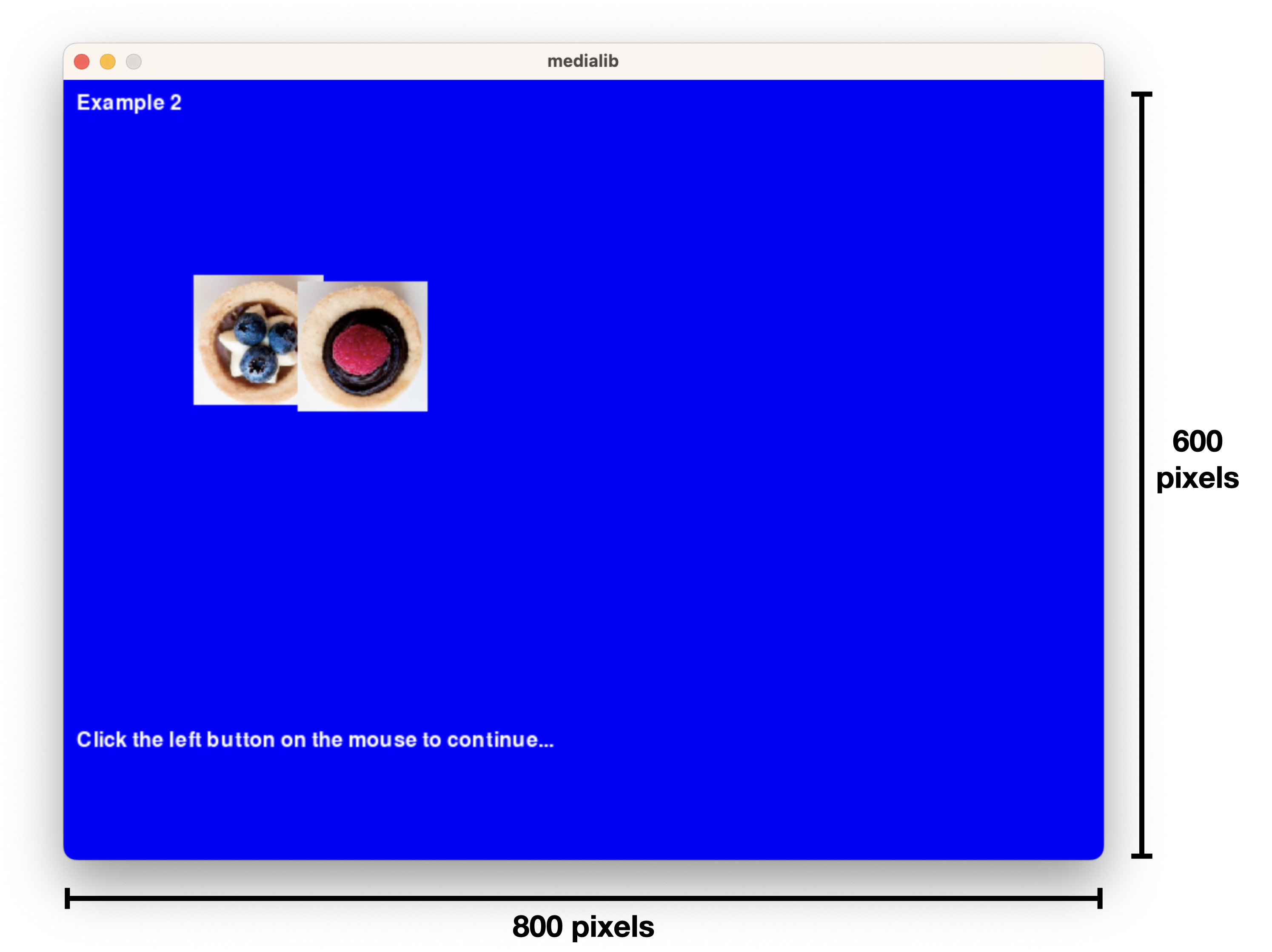Remember to “stop” the program after you are done.

###### Anatomy of Python commands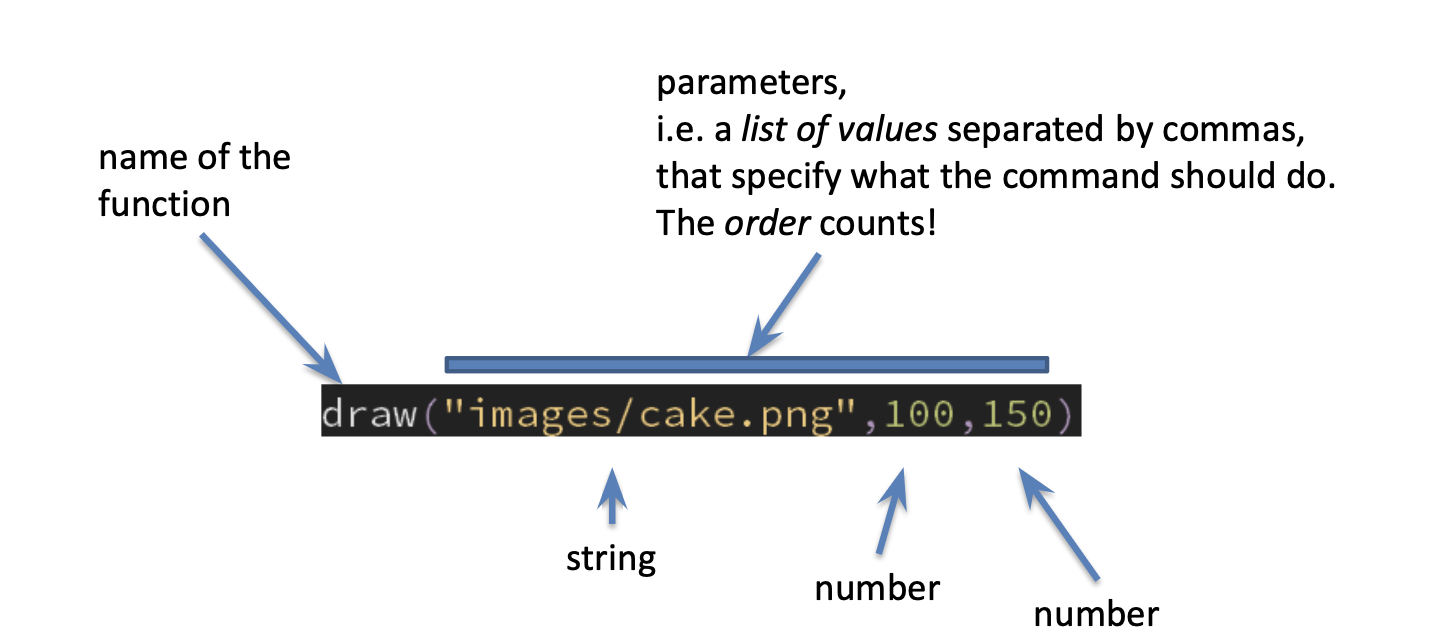If you look at the documentation, you will see the names for the parameters: e.g. `draw(imgFileName,x,y)`. The names explain what the parameters are or do.

###### The coordinate system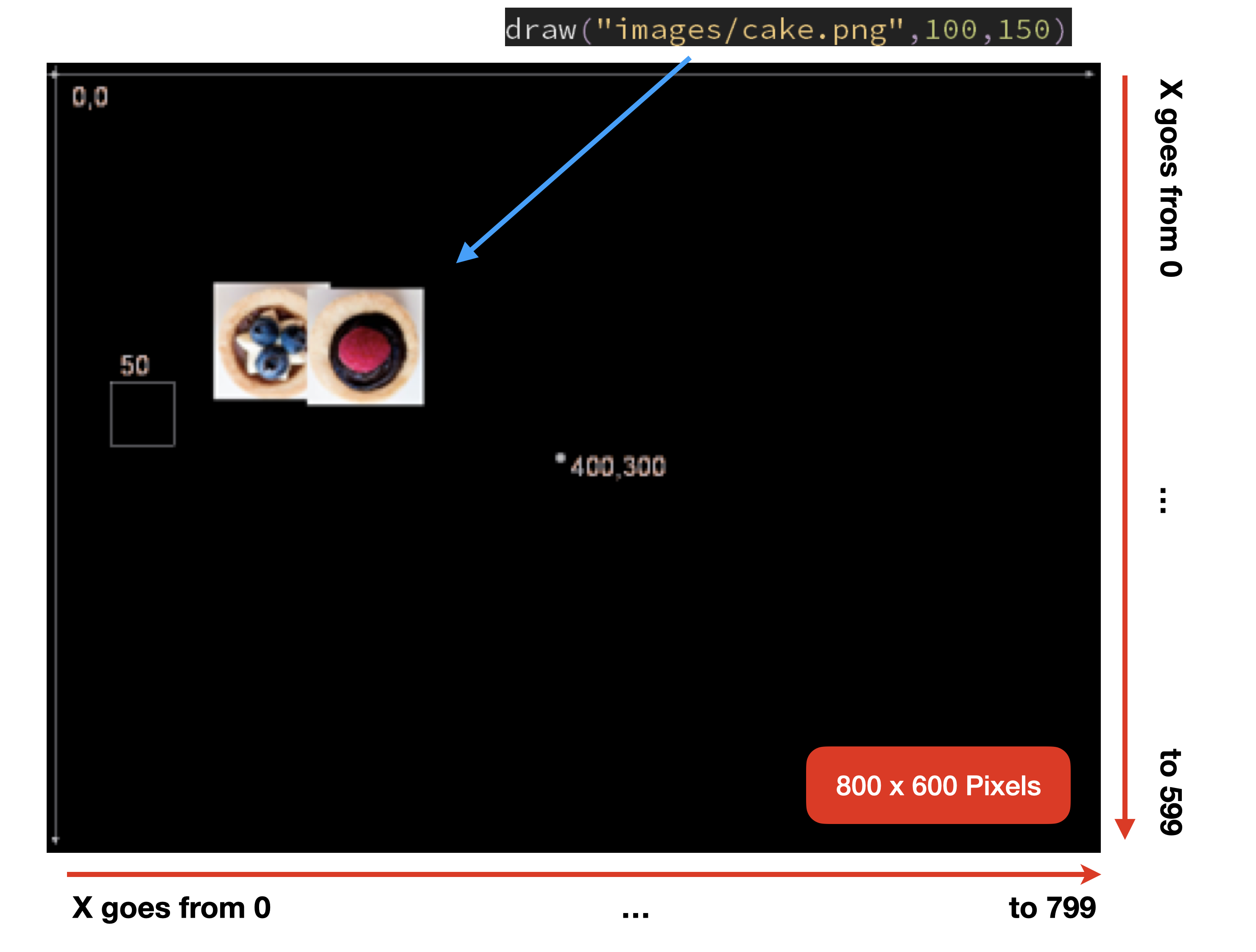• `import`
• is for using a library, code written by someone else. Here we want `import medialib.py` so we can use the commands in that program, to draw on screen (for example).
• `draw`
• given the name of an image file, and 2 numbers (X and Y coordinates), draws the image at that position. The top-left corner of the image will be at point (x,y).
•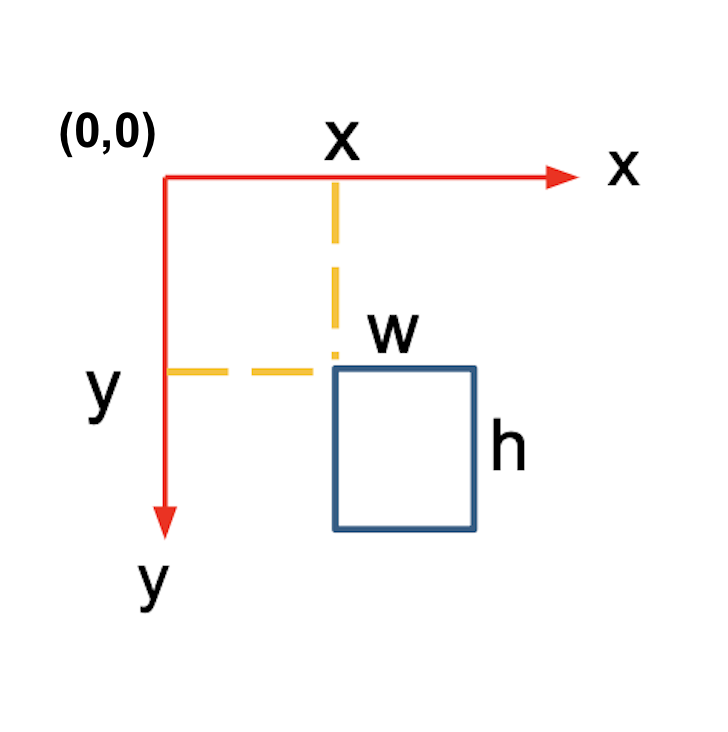• `wait_mouse_leftclick()`
• blocks the program until the user presses the left mouse button.
• `text`
• takes a message, 2 numbers (i.e. coordinates) and the size of the font, and writes the message at the given coordinates.
• `clear`
• Clears the drawing window. The default background color is black.
• `all_done`
• Don’t forget to have this command at the of all your programs that use the medialib. This command closes the graphic window.

See the documentation for more detailed information.

##### Exercises
###### Exercise 1

Copy and paste all the code from example.ipynb/example.py into the new file (CTRL+C then CTRL+V in the new file) then save the new program with name: morecakes.ipynb/morecakes.py

Now: modify the program in morecakes.ipynb/py:

• so that the 2 cakes are side by side, and with more space between them, like this: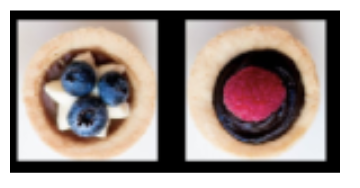• and place another cake (strawberry_cake.png) at another position on the screen. Can you place it in the center?
###### Exercise 2

Open the file practice1_for learner.ipynb.1. Move both eye.png and eye2.png 50 pixels downwards and pause the control flow of the program until the mouse's left button is pressed.
2.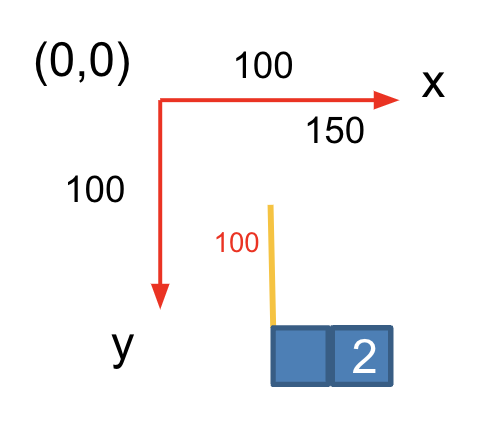3. Move both eye.png and eye2.png 50 pixels going towards the right and pause the control flow of the program until the mouse's left button is pressed.
4.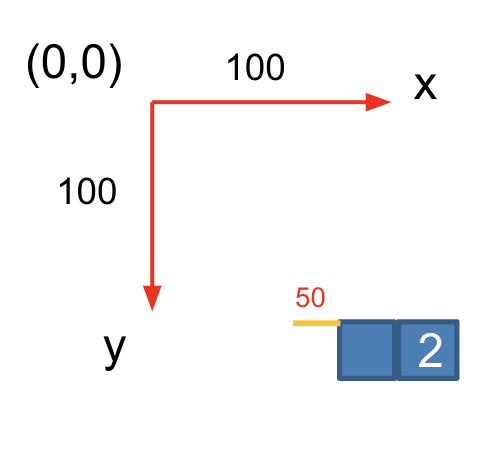5. Use variables to make the code more readable.
6. Try to define a function yourself to further improve the readability of the previous code.
7. Try to change `print()` to `text()` and see what happens.

#### Practice 1-2

##### Learning Objectives

We are going to learn:

1. How to use variables to make the code more readable.
2. How to define a function yourself.
3. How to define a fruitful function.
4. How to modify a fruitful function when the requirement changes.
##### The rect() function

`rect(x,y,w,h,r=None,g=None,b=None)`

Similar to `draw(...)`. This function draws a rectangle from the point `(x,y)` to the point `(x+w,y+h)`.

By default the rectangle border and inner area are white, but a color can be specified (in RGB format).

Example: `rect(10,15,100,120)`

Reminder: the top-left corner of the screen is at (0,0).##### Exercises
1. Write a program using rect() such that each time the mouse is left-clicked, the rectangle moves to the right by 100 pixels and becomes wider. Let `beginningXPosition=150`, `beginningYPosition=100`, `length=10` and `width=10`.
2.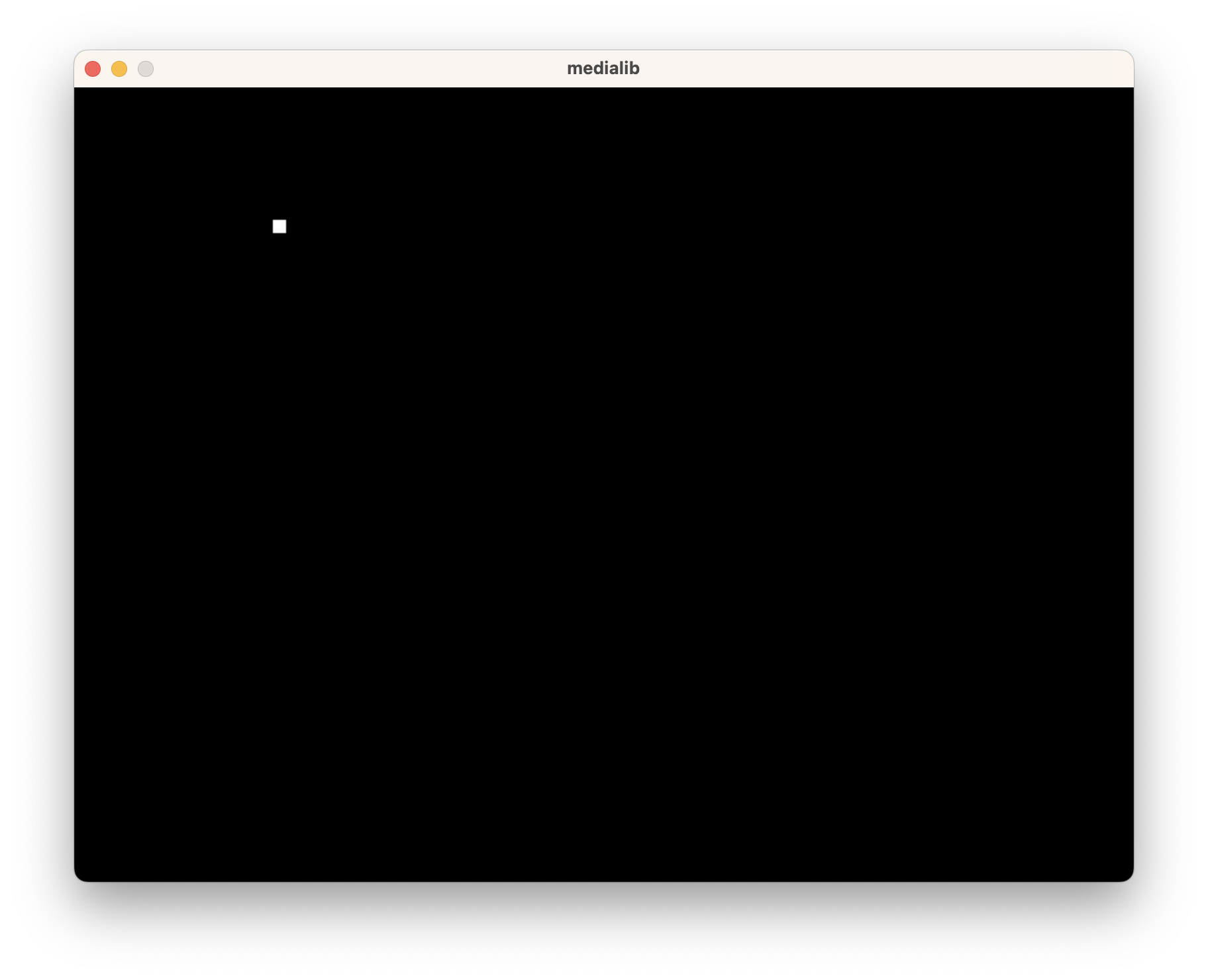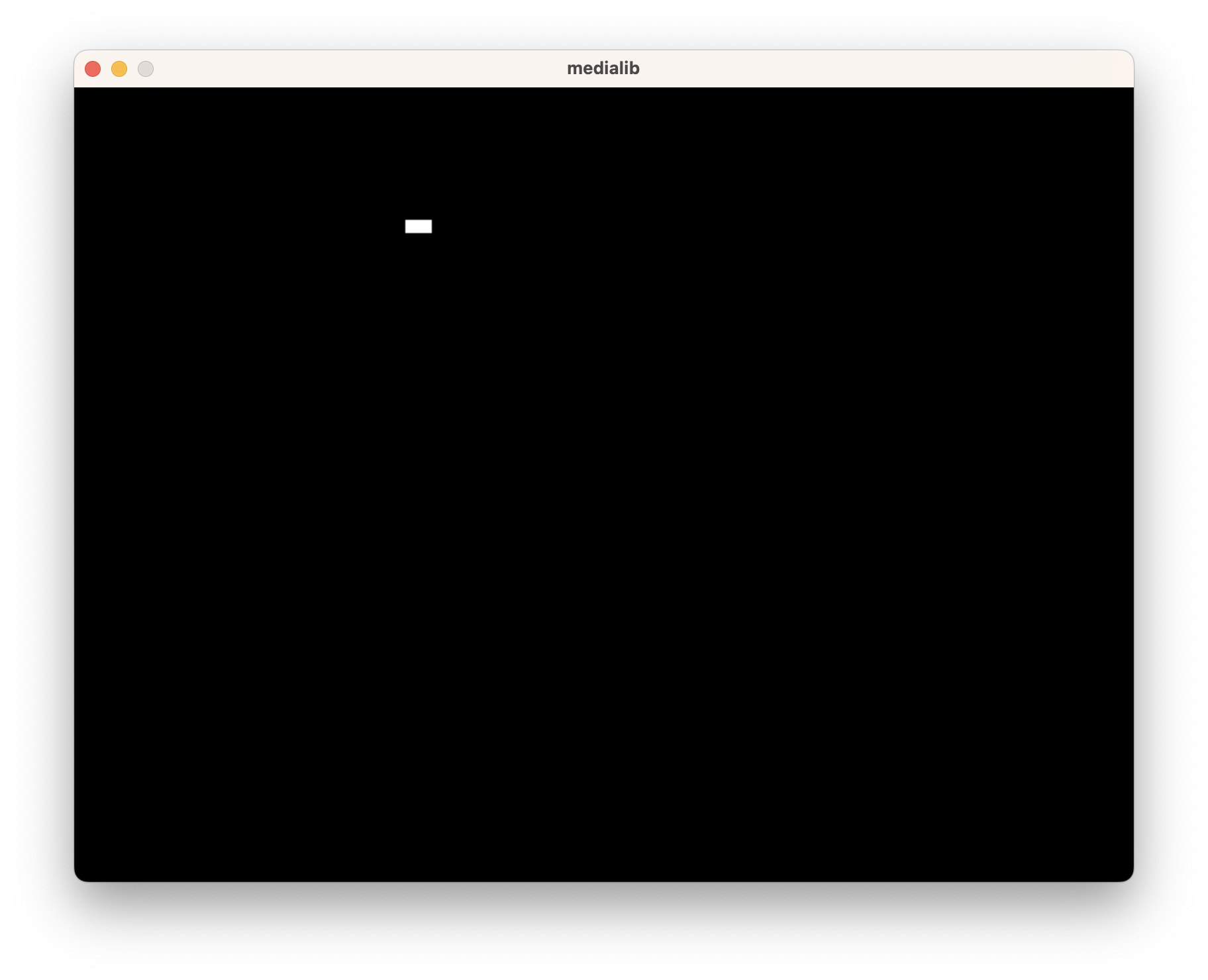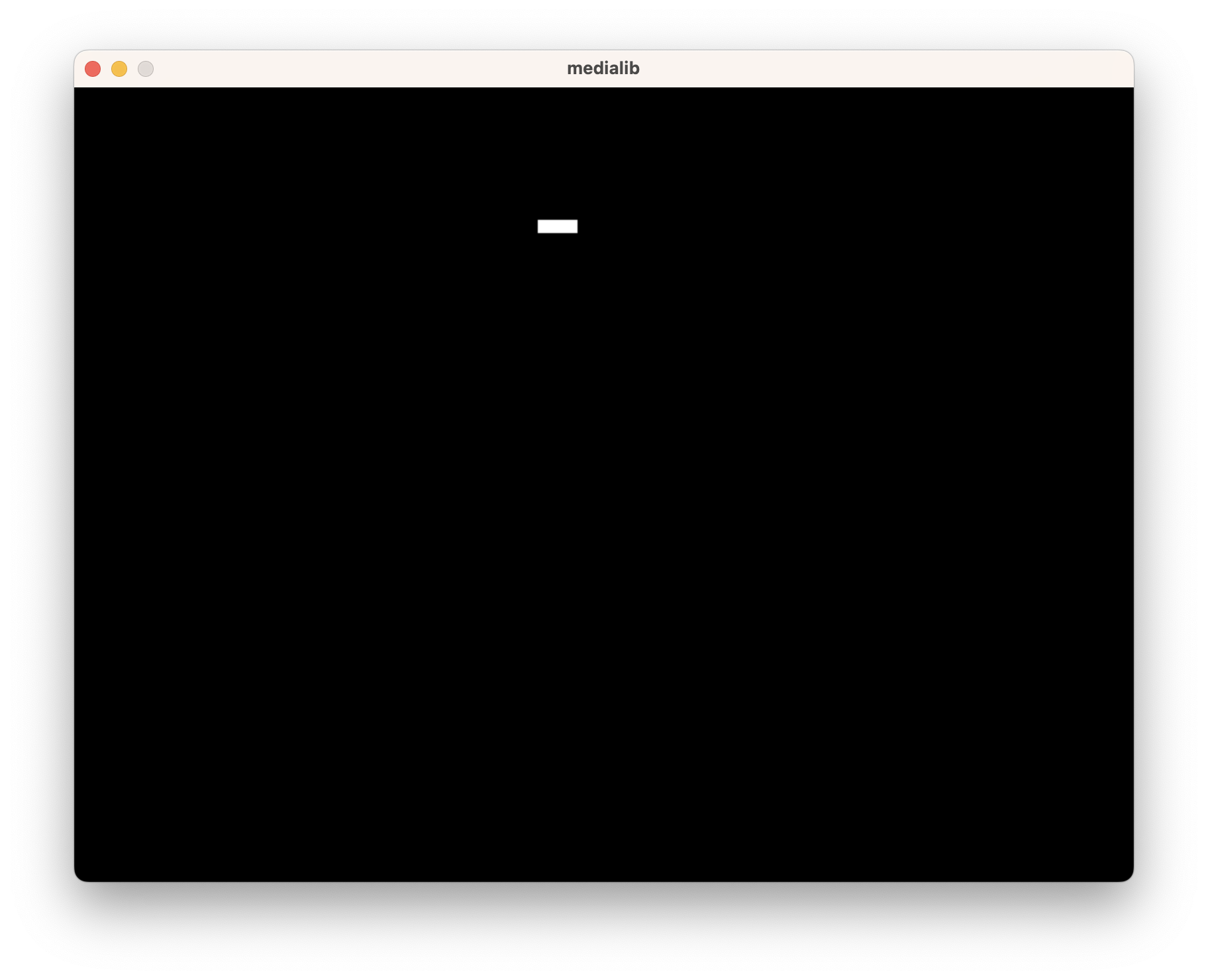3. Write another program using rect() such that each time the mouse is left-clicked, the rectangle moves down by 100 pixels and becomes wider. Let `beginningXPosition=150`, `beginningYPosition=100`, `length=10` and `width=10`.
4.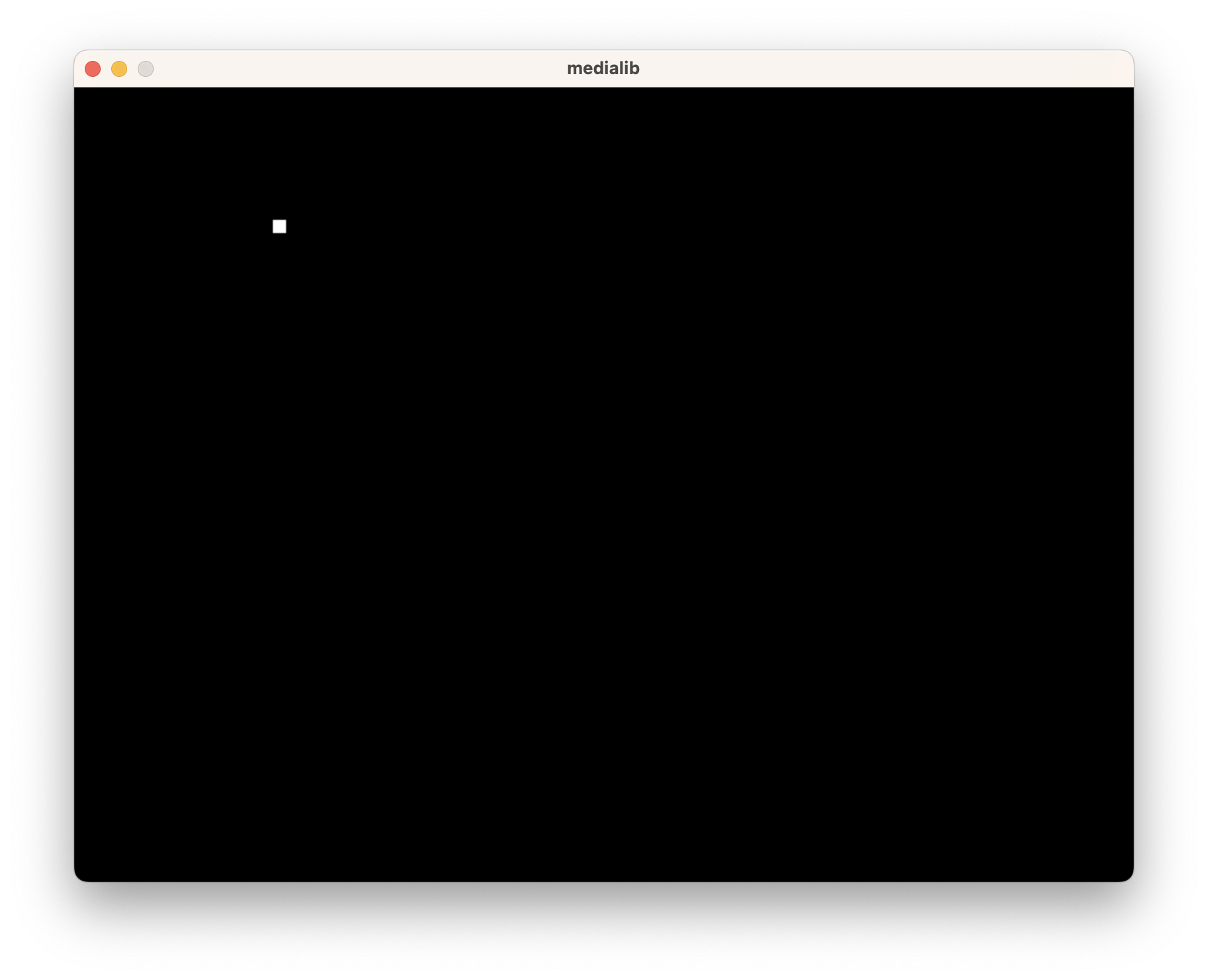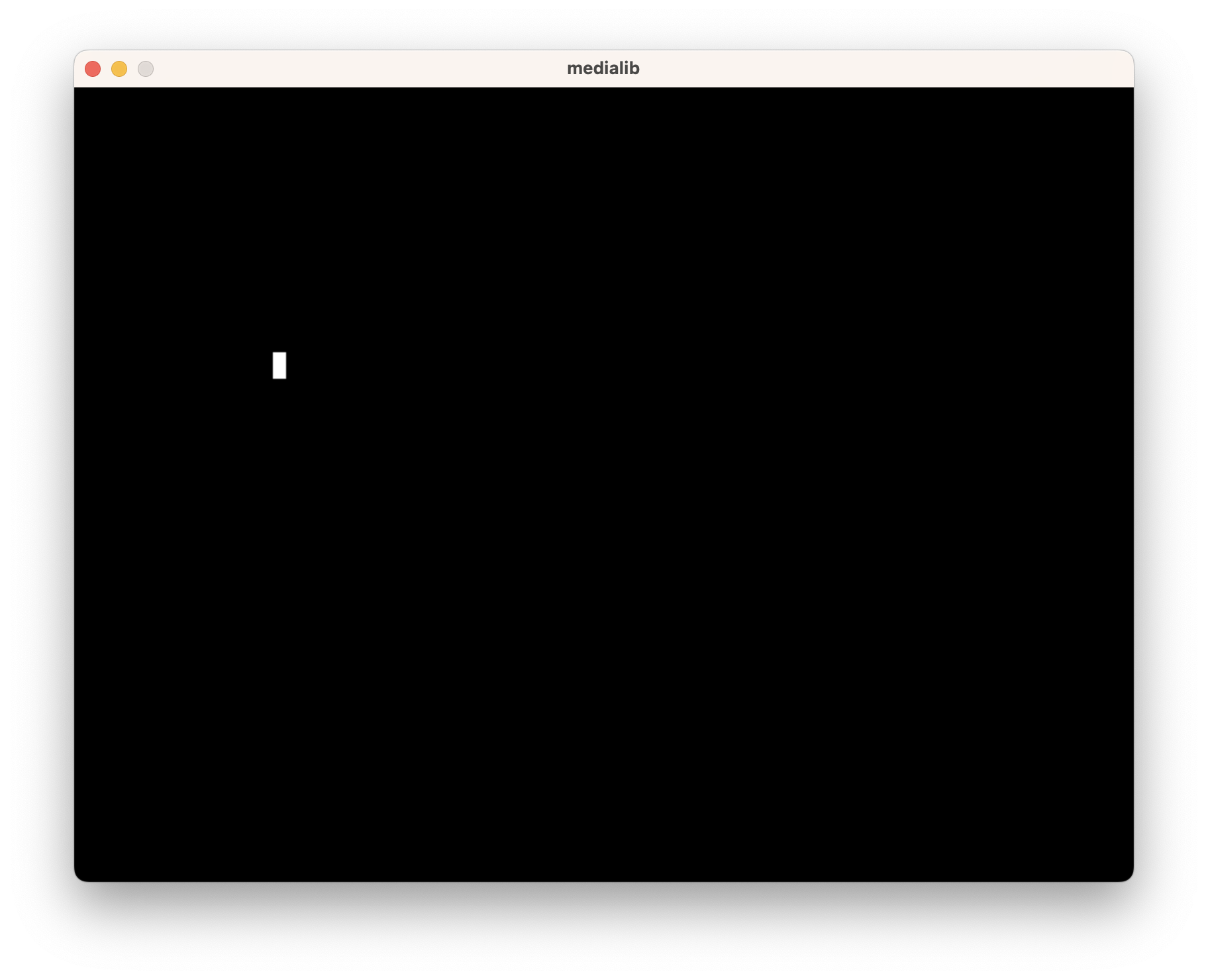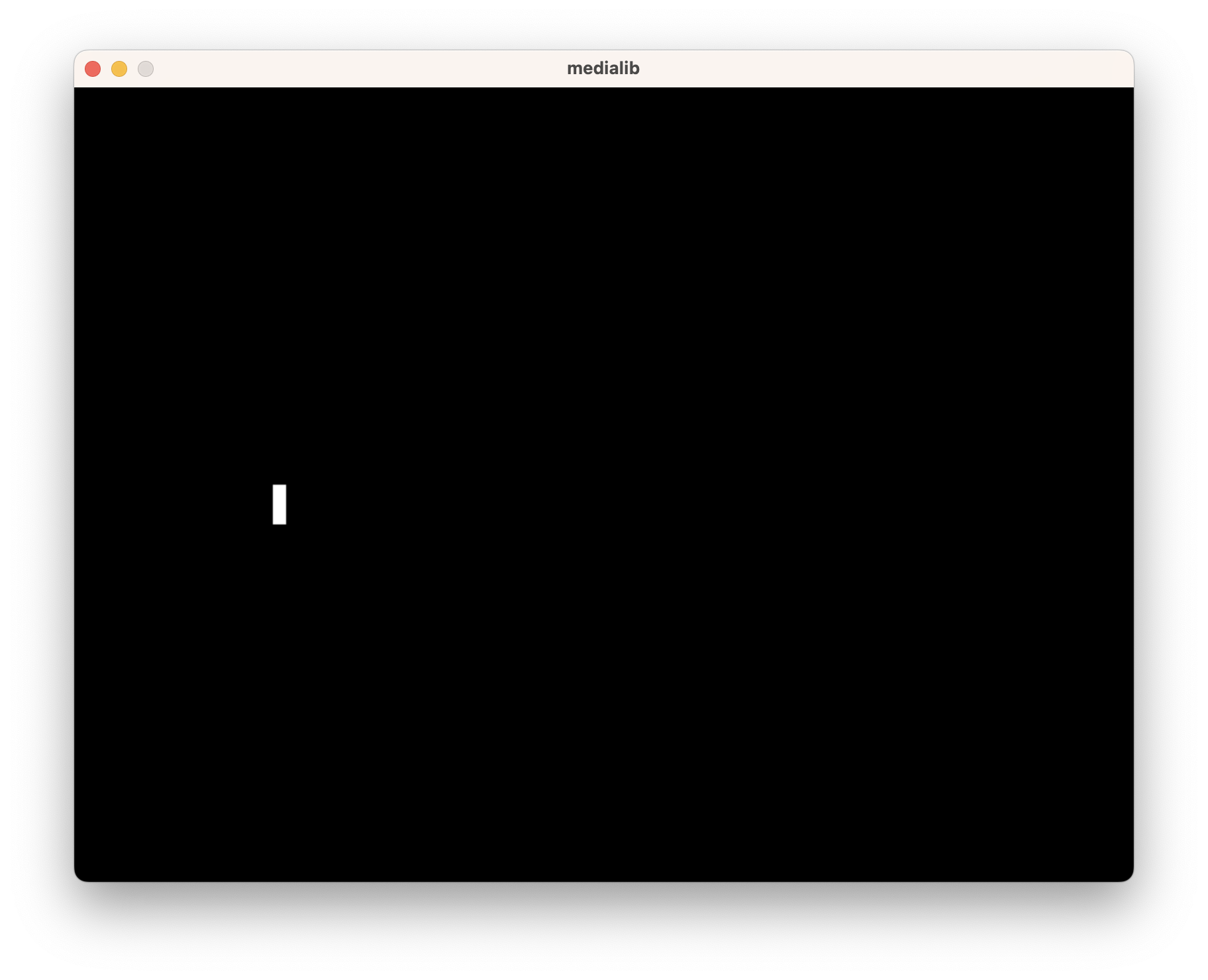### Practice 2

#### Practice 2-1

##### Learning Objectives

We are going to learn:

1. How to use `if`
2. How to use `while`
##### The play() and wait_key_press() function
###### The play() function

`play(soundFileName)`

Loads the audio file with name `soundFileName` and plays it.

If the audio file cannot be loaded then no audio will be played and an error message will be printed in the Python console. No exceptions are thrown, and the program will not break even if the audio file is not found.

###### The wait_key_press() function

`wait_key_press()`

Pauses the program until a key is pressed (any key).

If ESC, a number or a character is pressed, it will return a character. Otherwise it will return a code.

Example:

``````k = wait_key_press()
if k=="a":
print("you pressed -a-")``````
##### Exercises
1. Draw the picture python_logo.png at (100,100).
2. If the 'a' key is pressed, move the image to the left.
3. If the 'd' key is pressed, move the image to the right.
4. If the 'h' key is pressed, play the file hello.mp3 which says "Hello!".
5. If the 'q' key is pressed, exit the program.

#### Practice 2-2

##### Learning Objectives

We are going to learn:

1. How to draw game backgrounds using strings
2. How to use `while` and `if`
3. How to use `for` to replace `while`
4. How to draw game backgrounds using lists
##### Drawing Game Background using Strings

To draw background, you can use strings as a parameter to a function.

`eg. `trees = " BM     B  B"` and `floor = "GGGWWWGGGWGG"`. `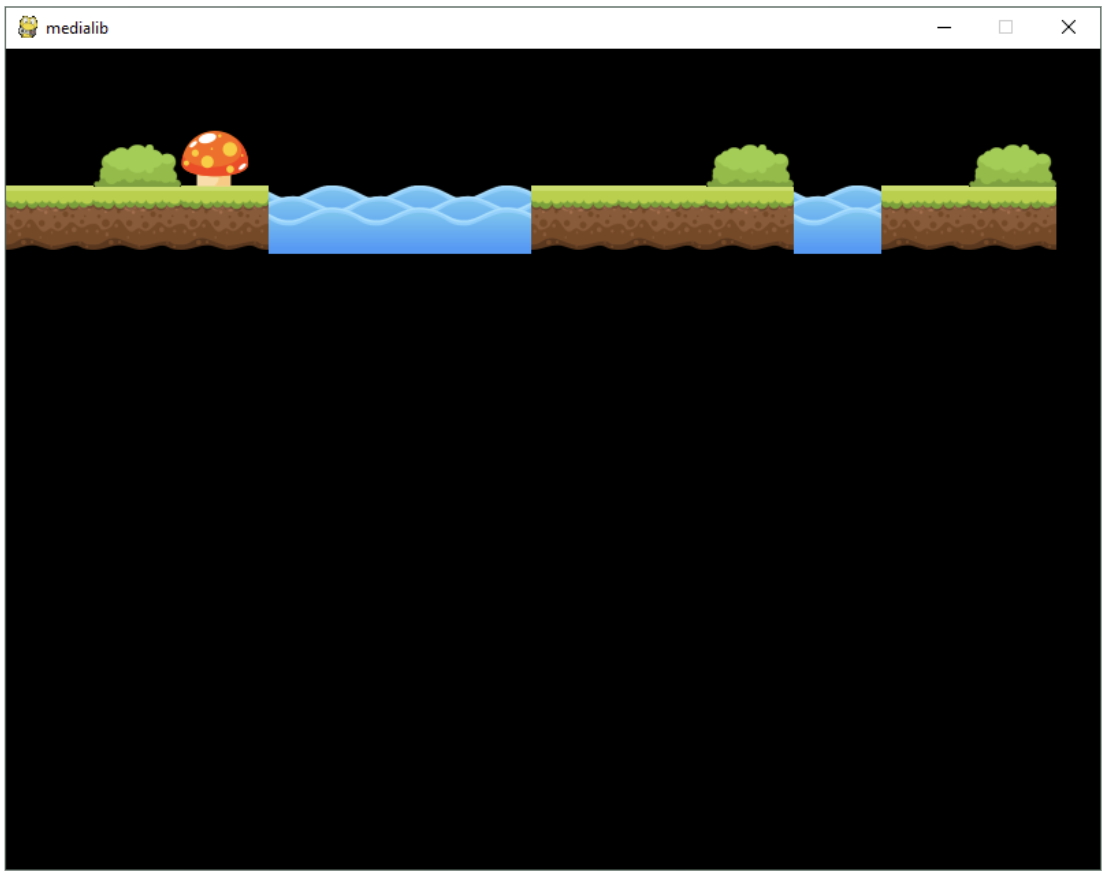##### Exercises
1. Finish the code below then change the tiles to have two more mushrooms.
2. Reverse the two strings so that the whole image becomes mirrored (as shown below).
3.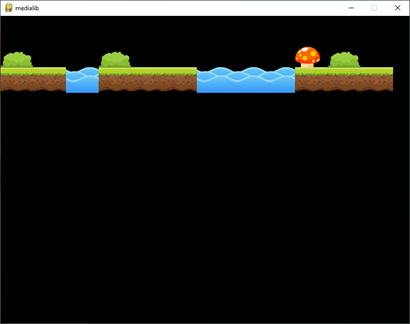4. What if we change lines 10 and 11 to `background=[[' ', 'B', 'M', ' ', ' ', ' ', ' ', ' ', 'B', ' ', ' ', 'B'],['G', 'G', 'G', 'W', 'W', 'W', 'G', 'G', 'G', 'W', 'G', 'G']]`

#### Extra Practice 1

##### Learning Objectives

We are going to learn:

1. How to use `for` and `range`
##### The wait() function

`wait(secs)`

This blocking command stops the control flow of the program for a certain number of seconds.

Real numbers can be used for the seconds.

Example:

`wait(1.5) ## pause the program for 1.5 seconds`

##### Exercise

Write a function using `for` and `range` to create an animation which draws a rectangle from big to small.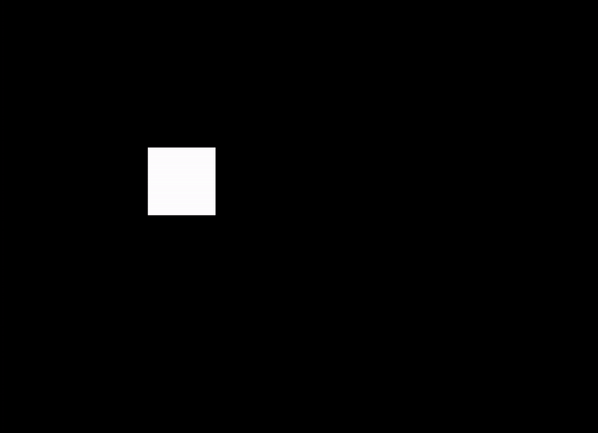#### Extra Practice 2

##### Learning Objectives

We are going to learn:

1. How to use `for` and `range`
2. How to make use of lists
##### Exercises

Draw a bar chart which consists of several horizontal bars where the length of each bar is stored in the list named 'values'.

1. Add a new element with the value '360' to the end of the list 'values'.
2. Let the height of the bar be 30, draw a bar chart which consists of several horizontal bars where the length of each bar is stored in the list named 'values'.
3. Try to organize the elements in values from small to big, and then draw the chart again.